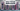# Find if Array contains Duplicate Number - Leet Code Solution

September 01, 2020## Problem Statement

Given an array of integers, find if the array contains any duplicates.

Your function should return true if any value appears at least twice in the array, and it should return false if every element is distinct.

Example

``````Input: [1,2,3,1]
Output: true``````

## Brute Force Solution

Lets talk about the simplest solution first.

• You can have two loops
• Outer loop iterate over complete array
• Inner loop covers rest of the array
• Just check if rest of the array contains same number or not

### Code

``````public boolean containsDuplicate_bruteforce(int[] nums) {
if (nums == null || nums.length == 0) return false;

int l = nums.length;
for (int i=0; i<l; i++) {
for (int j=i+1; j<l; j++) {
if (nums[i] == nums[j]) {
return true;
}
}
}
return false;
}``````

### Complexity

Its `O(n^2)`

## Another Solution - Sorting

Another simple solution is to sort the numbers first. Then, match consecutive numbers. If you find any pair having same value, return true.

### Code

``````public boolean containsDuplicate(int[] nums) {
if (nums == null || nums.length == 0) return false;

Arrays.sort(nums);
int l = nums.length;
for (int i=1; i<l; i++) {
if (nums[i-1] == nums[i]) {
return true;
}
}
return false;
}``````

### Complexity

Complexity for sorting is `O(nlogn)` Total complexity is also `O(nlogn)`

## Another Solution using Extra Memory - HashSet

Lets utilize a HashSet. A HashSet is a data structure which contains all unique elements, and the complexity to search and adding an element is `O(1)`

``````public boolean containsDuplicate_extraMemory(int[] nums) {
if (nums == null || nums.length == 0) return false;

Set<Integer> set = new HashSet<>();
int l = nums.length;
for (int i=0; i<l; i++) {
if (set.contains(nums[i])) {
return true;
}
Its `O(n)`